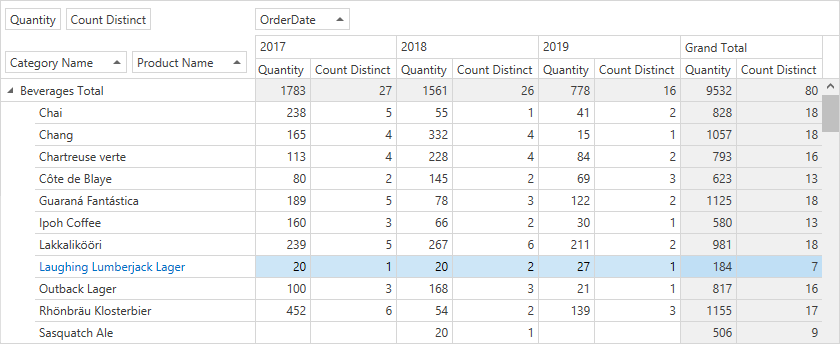# ExpressionDataBinding Class

Defines a calculation based on a string expression.

Namespace: DevExpress.Xpf.PivotGrid

Assembly: DevExpress.Xpf.PivotGrid.v22.2.dll

NuGet Package: DevExpress.Wpf.PivotGrid

## Declaration

``````public class ExpressionDataBinding :
DataBinding,
IExpressionBinding``````

## Remarks

Calculated fields display the result of calculated expressions. You can use a formula or an aggregate function as a binding expression for each calculated field. The expression allows you to not only obtain values from a field in the data source, but also specify exactly how to calculate the data (for example, aggregate it).

Note

Use OlapExpressionBinding in OLAP mode.

Follow the steps below to create a calculated field in Optimized and Server modes:

1. Create an `ExpressionDataBinding` instance and pass the expression in its constructor as a parameter.
2. Assign the created object to the PivotGridField.DataBinding property.

The example below shows how create a `Count Distinct` field and bind it to an expression that contains a custom function. The expression counts distinct values (the number of orders with equal product quantities).``````<dx:ThemedWindow x:Class="DXPivotGrid_CustomSummary.MainWindow" mc:Ignorable="d" Title="MainWindow" Height="450" Width="800"
xmlns:dx="http://schemas.devexpress.com/winfx/2008/xaml/core"
xmlns="http://schemas.microsoft.com/winfx/2006/xaml/presentation"
xmlns:x="http://schemas.microsoft.com/winfx/2006/xaml"
xmlns:d="http://schemas.microsoft.com/expression/blend/2008"
xmlns:mc="http://schemas.openxmlformats.org/markup-compatibility/2006"
xmlns:local="clr-namespace:DXPivotGrid_CustomSummary"
xmlns:dxpg="http://schemas.devexpress.com/winfx/2008/xaml/pivotgrid">
<Grid>

DataProcessingEngine="Optimized">
<dxpg:PivotGridControl.Fields>
<!-- ... -->
<dxpg:PivotGridField Name="fieldQuantityDistinctCount"  Area="DataArea" AreaIndex="1"
Caption="Count Distinct">
<dxpg:PivotGridField.DataBinding>
<dxpg:ExpressionDataBinding Expression="DistinctCount([OrderID])"/>
</dxpg:PivotGridField.DataBinding>
</dxpg:PivotGridField>
</dxpg:PivotGridControl.Fields>
</dxpg:PivotGridControl>

</Grid>
</dx:ThemedWindow>
``````

The following code snippets (auto-collected from DevExpress Examples) contain references to the ExpressionDataBinding class.

Note

The algorithm used to collect these code examples remains a work in progress. Accordingly, the links and snippets below may produce inaccurate results. If you encounter an issue with code examples below, please use the feedback form on this page to report the issue.